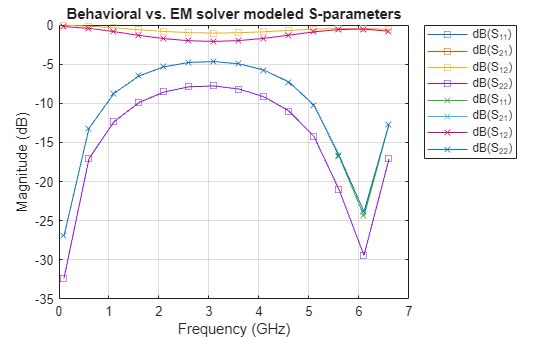# txlineCPW

Create coplanar waveguide transmission line

## Description

Use the `txlineCPW` object to create a coplanar waveguide (CPW) transmission line. You can also use the `txlineCPW` object to create an infinite-bottom conductor or ground plane CPW transmission line. The cross-section of a CPW transmission line is shown in this figure. The physical characteristics of the CPW transmission line include conductor width (w), conductor thickness (t), slot width (s), substrate height (d), and permittivity constant (ε).## Creation

### Syntax

``txline = txlineCPW``
``txline = txlineCPW(Name,Value)``

### Description

````txline = txlineCPW` creates a default CPW transmission line object.```

example

````txline = txlineCPW(Name,Value)` sets Properties using one or more name-value pairs. For example, `txline = txlineCPW('SlotWidth',0.0046)` creates a CPW transmission line with a slot width of `0.0046` meters.```

## Properties

expand all

Name of the CPW transmission line, specified as a string scalar or a character vector.

Example: `'Name','cpw1'`

Example: `txline.Name = 'cpw1'`

Data Types: `char` | `string`

Physical width of the conductor, specified as a positive scalar in meters.

Example: `'ConductorWidth',0.0200`

Example: `txline.ConductorWidth = 0.0200`

Data Types: `double`

Physical width of the slot, specified as a positive scalar in meters.

Example: `'SlotWidth',0.0008`

Example: `txline.SlotWidth = 0.0008`

Data Types: `double`

Physical height of the conductor or dielectric thickness, specified as a positive scalar in meters.

Example: `'Height',0.000835`

Example: `txline.Height = 0.000835`

Data Types: `double`

Physical thickness of the CPW transmission line, specified as a positive scalar in meters.

Example: `'Thickness',0.000008`

Example: `txline Thickness = 0.000008`

Data Types: `double`

Relative permittivity of the dielectric, specified as a positive scalar.

Example: `'EpsilonR',8.8`

Example: `txline.EpsilonR = 8.8`

Data Types: `double`

Loss angle tangent of the dielectric, specified as a nonnegative scalar.

Example: `'LossTangent',1`

Example: `txline.LossTangent = 1`

Data Types: `double`

Conductivity of the conductor, specified as a nonnegative scalar in Siemens per meter (S/m).

Example: `'SigmaCond',2`

Example: `txline.SigmaCond = 2`

Data Types: `double`

Physical length of the CPW transmission line, specified as a positive scalar in meters.

Example: `'LineLength',0.0200`

Example: `txline.LineLength = 0.0200`

Data Types: `double`

Infinite-bottom conductor or ground plane, specified as a numeric or logical. When you specify `0`, you create a transmission line without any conductor backing.

Example: `'ConductorBacked',1`

Example: `txline.ConductorBacked = 1`

Data Types: `double`

Stub transmission line termination, specified as `'NotApplicable'`, `'Open'`, or `'Short'`.

Example: `'Termination','Short'`

Example: `txline.Termination = 'Short'`

Data Types: `char`

Type of stub, specified as `'NotAStub'`, `'Series'`, or `'Shunt'`.

Example: `'StubMode','Series'`

Example: `txline.StubMode = 'Series'`

Data Types: `char`

Number of input and output ports, returned as positive scalar.

Data Types: `double`

Terminals of the CPW transmission line, returned as a cell array of strings.

Data Types: `char` | `string`

## Object Functions

 `sparameters` Calculate S-parameters for RF data, network, circuit, and matching network objects `groupdelay` Group delay of S-parameter object or RF filter object or RF Toolbox circuit object `noisefigure` Calculate noise figure of transmission lines, series RLC, and shunt RLC circuits `getZ0` Calculate characteristic impedance with and without dispersion for transmission line `circuit` Circuit object `clone` Create copy of existing circuit element or circuit object

## Examples

collapse all

Create a coplanar waveguide transmission line using these specifications:

• Conductor width : 45 mu m

• Slot width : 50 mu m

• Height of substrate : 525 mu m

• Thickness : 1 mu m

• GaAS permittivity or Epsilon R : 2.5 F/m

• Conductivity : 3.33e7 S/m

```cpwtxline = txlineCPW('ConductorWidth',45e-6,'SlotWidth',50e-6,'Height',525e-6,... 'Thickness',1e-6,'EpsilonR',2.5,'SigmaCond',3.33e7)```
```cpwtxline = txlineCPW: CPW element Name: 'CPW' ConductorWidth: 4.5000e-05 SlotWidth: 5.0000e-05 Height: 5.2500e-04 Thickness: 1.0000e-06 EpsilonR: 2.5000 LossTangent: 0 SigmaCond: 33300000 LineLength: 0.0100 ConductorBacked: 0 Termination: 'NotApplicable' StubMode: 'NotAStub' NumPorts: 2 Terminals: {'p1+' 'p2+' 'p1-' 'p2-'} ```

Calculate the S-parameters of the transmission line at 20 GHz.

`sparam = sparameters(cpwtxline,20e9);`

This example uses RF PCB Toolbox to calculate an electromagnetic (EM) solver S-parameter model of a conductor-backed coplanar waveguide (CPW) transmission line.

Behavioral Modeling

Select the FR4 dielectric and silver metal the metal and dielectric libraries, respectively, of RF PCB Toolbox.

```dFR4 = dielectric('FR4'); mSilver = metal('Silver');```

Design an infinite-bottom conductor-backed CPW transmission line at `6` GHz and ${\mathit{Z}}_{0}$ of `75`$\Omega$.

```tx = txlineCPW('ConductorBacked',true, ... 'LineLength',0.0145,'ConductorWidth',2.6482e-4, ... 'SlotWidth',2e-4,'Height',6.35e-4, ... "EpsilonR",dFR4.EpsilonR,"LossTangent",dFR4.LossTangent, ... "SigmaCond",mSilver.Conductivity,'Thickness',2e-7, ... 'StubMode',"NotAStub","Termination","NotApplicable");```

Calculate the S-parameters using a behavioral model from RF Toolbox with the reference impedance of `50`$\Omega$. The behavioral model is also called an analytical or closed-form model.

```freq = (1:5:66)*100e6; Srf = sparameters(tx,freq,50);```

EM Modeling

Create an RF PCB Toolbox `coplanarWaveguide` object.

`tx_em = coplanarWaveguide(tx);`

View the conductor-backed CPW transmission line.

`show(tx_em)`Calculate the S-parameters using the EM solver from RF PCB Toolbox.

`Sem = sparameters(tx_em,freq,50);`

Compare S-parameters

Compare the S-parameters from the behavioral and EM solver models.

```rfplot(Srf,'-s','db') hold on rfplot(Sem,'-x','db') title('Behavioral vs. EM solver modeled S-parameters')```Garg, Ramesh, I. J. Bahl, and Maurizio Bozzi. Microstrip Lines and Slotlines . 3rd ed. Artech House Microwave Library. Boston: Artech House, 2013.